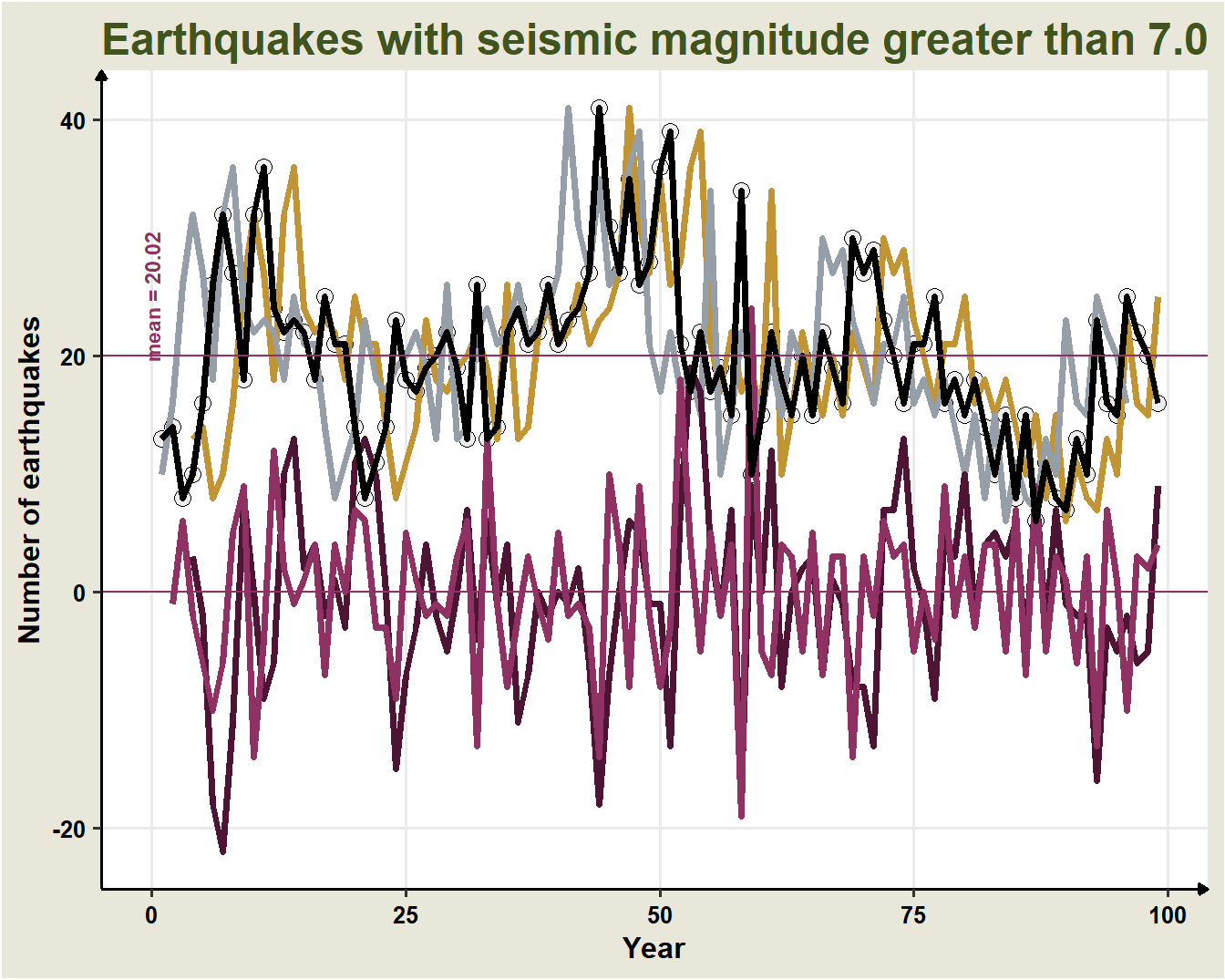## 17.4 Operator $$B$$

### 17.4.1 Lag operator

Lag Operator

Represented by $$B$$ or $$L$$, from Backshift operator o Lag operator.

$B Y_{t} = Y_{t-1s}$ where $$Y_{t}$$ ia a time series at time $$t$$

• $$B$$ indicates that the value at one instant is replaced by the value at the immediately preceding instant.

$B Y_{t} \equiv B(Y_{t-1}) \equiv B:Y_{t-1}$

• A double application of $$B$$ is denoted by:

$B^{2} Y_{t}=B\left(B Y_{t}\right)=B Y_{t-1}=Y_{t-2}$

• In general,

$B^{d} Y_{t} = Y_{t-d}$

### 17.4.2 Difference operator

Operator Difference

Represented by the symbol $$\nabla$$

$\nabla Y_{t} = Y_{t} - Y_{t-1}$ where $$Y_{t}$$ is a time series at time $$t$$

Examples:

\begin{aligned} Y_{1}-Y_{0} &=\nabla Y_{1}\\ Y_{2}-Y_{1} &=\nabla Y_{2}\\ Y_{n}-Y_{n-1} &=\nabla Y_{n} \end{aligned} The regular difference of order d operator ( $$d\geq 1$$ integer) is represented by $$\nabla^{d}$$ (or $$\Delta^d$$) and denotes the successive application of $$\nabla:$$

$\nabla^{d} Y_{t} = (1-B)^d Y_{t}$

For example:

\begin{aligned} \nabla^{2} Y_{t} &=(1-B)^{2} Y_{t}=\left(1-2 B-B^{2}\right) Y_{t} \\ \nabla^{3} Y_{t} &=Y_{t}-3 B Y_{t}+3 B^{2} Y_{t}-B^{3} Y_{t} \end{aligned}

$$\nabla^{s}$$ denotes the seasonal difference defined by:

$\nabla^{s} Y_{t} = (1-B^s) Y_{t} = Y_t - Y_{t-12}$

### 17.4.3 Forward operator

Forward operator

Represented by the symbol $$B^-$$ or $$F$$

$B^{-1}Y_{t} = Y_{t+1}$ where $$Y_{t}$$ is a time series at time $$t$$.

* F\$ is the lag operator used in the inverse direction, i.e. $$d<0$$, a new series will be ahead by $$d$$ time units. * $$B^{-1}$$ is the lead operator * In general, $B^{-d} Y_{t} \equiv B^{-d}(Y_{t}) = Y_{t+d}$

### 17.4.4 Properties of $$B$$

• The delay of a constant is a constant, $$c$$:

$Bc=c$

• Distributive property:

$\left(B^{i}+B^{j}\right) Y_{t}=B^{i} Y_{t}+B^{j} Y_{t}=Y_{t-i}-Y_{t-j}$

• Associative property:

\begin{aligned} B^{i} B^{j} X_{t}&=B^{i}\left(B^{j} Y_{t}\right)=B^{i} Y_{t-j}=Y_{t-i-j}\\ B^{i} B^{j} X_{t}&=B^{i+j} y_{t}, \text { y } \\ B^{0} X_{t}&=X_{t} \end{aligned}

• If $$|a| < 1,$$

$\left(1+a B+a^{2} B^{2}+a^{3} B^{3}+\ldots\right) Y_{t}=\frac{Y_{t}}{(1-a B)}$

### 17.4.5 Equations in Difference

• A difference equation expresses the value of a variable as a function of its own lagged values, time and other variables.
• The $$B$$ operator can be used to write compact difference equations:

\begin{aligned} Y_{t} &=a_{0}+a_{1} Y_{t-1}+\ldots+a_{p} Y_{t-p}+\varepsilon_{t} \\ \left(1-a_{1} B-a_{2} B^{2}-\ldots-a_{p} B^{p}\right) Y_{t} &=a_{0}+\varepsilon_{t}\\ \Rightarrow A(B) X_{t}&=a_{0}+\varepsilon_{t} \end{aligned}

where $$A(B)$$ can be viewed as a polynomial of the operator $$B.$$

• Another example:

\begin{aligned} X_{t} &=a_{0}+a_{1} X_{t-1}+\ldots+a_{p} X_{t-p}+\varepsilon_{t}+\beta_{1} \varepsilon_{t-1}+\ldots+\beta_{q} \varepsilon_{t-q} \\ A(B) X_{t} &=a_{0}+M(B) \varepsilon_{t} \end{aligned}

The $$B$$ operator can be used to solve equations in differences:

$Y_{t} = a_{0}+a_{1} Y_{t-1}+\varepsilon_{t} \\ Y_{t} = a_{0}+B Y_{t}+\varepsilon_{t} \\ Y_{t} = \frac{a_{0}+\varepsilon_{t}}{1-a_{1} B}$

### 17.4.6 Example:

Applying the operator $$B$$ to the series of earthquakes:

Earthquakes: First 10 data points
$$t$$ $$Y_t$$ $$BY_{t}$$ $$B^3Y_{t}$$ $$B^{-1}Y_{t}$$ $$B^{-1}Y_{t}$$ $$\nabla Y_{t}$$ $$\nabla^{3}Y{t}$$
1 13 NA NA 14 10 NA NA
2 14 13 NA 8 16 -1 NA
3 8 14 NA 10 26 6 NA
4 10 8 13 16 32 -2 3
5 16 10 14 26 27 -6 -2
6 26 16 8 32 18 -10 -18
7 32 26 10 27 32 -6 -22
8 27 32 16 18 36 5 -11
9 18 27 26 32 24 9 8
10 32 18 32 36 22 -14 0